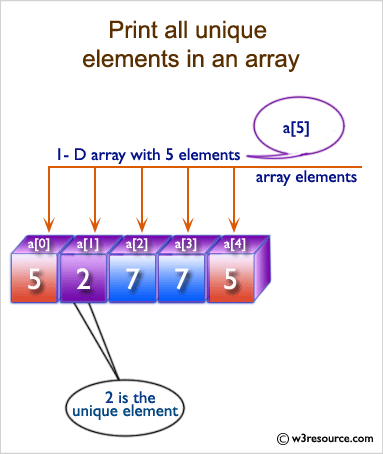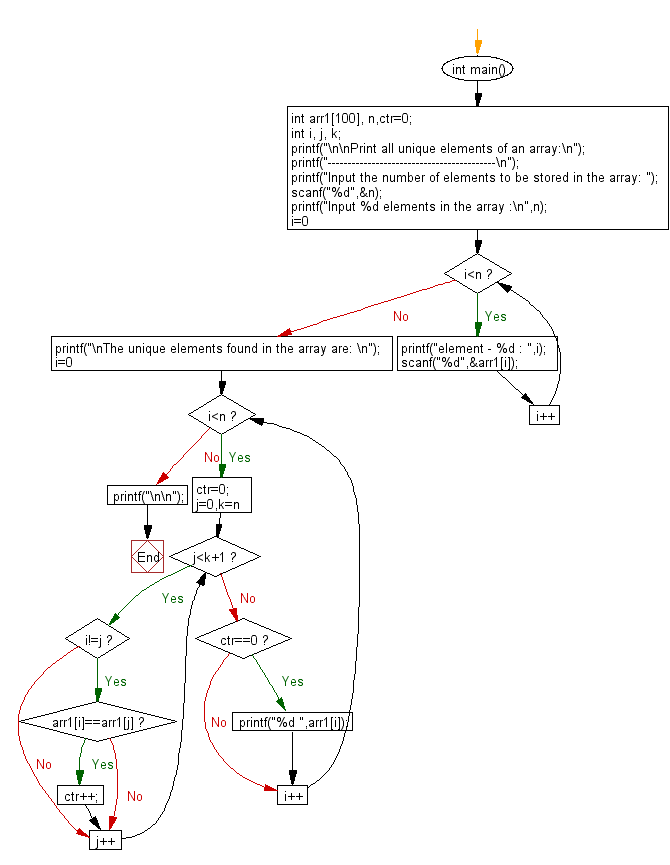﻿ C exercises: Print all unique elements of an array - w3resource# C Exercises: Print all unique elements of an array

## C Array: Exercise-6 with Solution

Write a program in C to print all unique elements in an array.

Pictorial Presentation:Sample Solution:

C Code:

``````#include <stdio.h>

void main()
{
int arr1, n,ctr=0;
int i, j, k;

printf("\n\nPrint all unique elements of an array:\n");
printf("------------------------------------------\n");

printf("Input the number of elements to be stored in the array :");
scanf("%d",&n);

printf("Input %d elements in the array :\n",n);
for(i=0;i<n;i++)
{
printf("element - %d : ",i);
scanf("%d",&arr1[i]);
}

/*Checking duplicate elements in the array */
printf("\nThe unique elements found in the array are : \n");
for(i=0; i<n; i++)
{
ctr=0;

/*Check duplicate bifore the current position and
increase counter by 1 if found.*/
for(j=0; j<i-1; j++)
{
/*Increment the counter when the seaarch value is duplicate.*/
if(arr1[i]==arr1[j])
{
ctr++;
}
}
/*Check duplicate after the current position and
increase counter by 1 if found.*/
for(k=i+1; k<n; k++)
{
/*Increment the counter when the seaarch value is duplicate.*/
if(arr1[i]==arr1[k])
{
ctr++;
}
}
/*Print the value of the current position of the array as unique value
when counter remain contains its initial value.*/
if(ctr==0)
{
printf("%d ",arr1[i]);
}
}
printf("\n\n");
}
```
```

Sample Output:

```Print all unique elements of an array:
------------------------------------------
Input the number of elements to be stored in the array :3
Input 3 elements in the array :
element - 0 : 1
element - 1 : 5
element - 2 : 1

The unique elements found in the array are :
5
```

Flowchart:C Programming Code Editor:

Improve this sample solution and post your code through Disqus.

What is the difficulty level of this exercise?

﻿